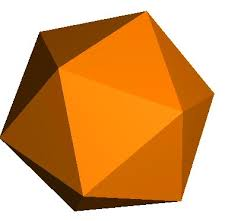# The lost bugA bug starts on a vertex and randomly moves along one edge of an icosahedron every 10 seconds to another vertex. What is the probability that it will end up at the opposite vertex after 30 seconds?

If the probability is expressed as $\dfrac ab$, where $a$ and $b$ are coprime positive integers, find $a+b$.

###### Try more questions on Platonic Solids.

Image credit: http://home.btconnect.com/

×